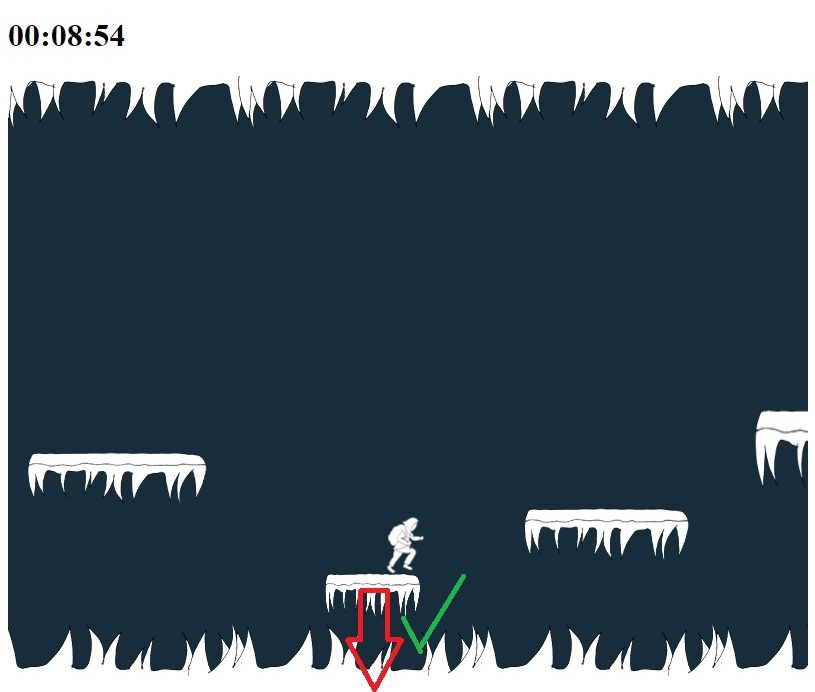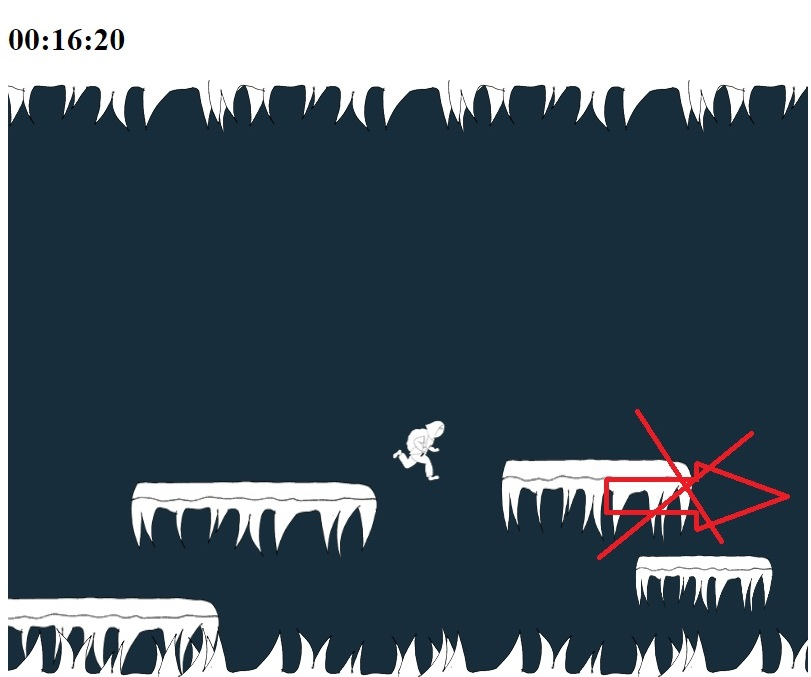# Get trapledges to fall in y direction only

## Recommended Posts

I thought this would be a simple fix, but so far can't figure it out at all. I have something I call trapledges that is going to fall in the y direction when colliding with player. However, I can not get them to stop moving in the x direction if the player touch the side of them. In my code I have a commented out if test in the update function. I've tried it out both there and in the else if test where the trapledges get made. also tried out different things in the test as changing velocity.x = 0; to for example gravity = 0; or trapLedge.immovable.x = true; and so on. Still won't stop floating to the side if the player touch the sides of the ledge, instead of landing ontop of it.

Added two jpgs of the game, with an arrow showing how the ledge move. A cross to show which way I want the ledge to stop move.

``````var playState = {

create: function() {

for (i = 0; i < 100; i++) {
var trapRandomizer = (Math.random() * 0.6) + 0.3;

var randomYPos = (Math.random() * 200) + 290;
var randomXSize = (Math.random() * 0.6) + 0.3;
var randomYSize =(Math.random() * 0.8) + 0.6;

//console.log("x: " + randomXSize + "Ran" + trapRandomizer)

if (trapRandomizer > 0.4) {
var ledge = platforms.create(ledgeXPos, randomYPos, "ground");
ledge.scale.setTo(randomXSize, randomYSize);
ledge.body.immovable = true;

}
else if (trapRandomizer <= 0.4) {
console.log("Trapledge");
var trapLedge = trapPlatforms.create(ledgeXPos, randomYPos, "ground");
trapLedge.scale.setTo(randomXSize, randomYSize);
trapLedge.body.immovable = false;
}

}

update: function() {

/*
trapPlatforms.body.velocity.x = 0;
trapPlatforms.body.velocity.y = 300;
}*/

},``````##### Share on other sites

Try any one of

• `trapLedge.body.maxVelocity.x = 0`
• `trapLedge.body.immovable = true `(this doesn't prevent all movement, only movement in response to `collide`)